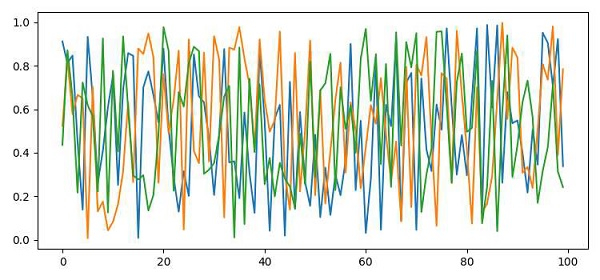# How to save the plot to a numpy array in RGB format?

To save the plot to a numpy array in RGB format, we can take the following steps −

• Create r, and random array using numpy.

• Zip r, and (grom step 1) to make an rgb tuple list.

• Convert rgb into a numpy array to plot it.

• Plot the numpy array that is in rgb format.

• Save the figure at the current location.

• To display the figure, use the show() method.

## Example

import numpy as np
from matplotlib import pyplot as plt
plt.rcParams["figure.figsize"] = [7.50, 3.50]
plt.rcParams["figure.autolayout"] = True
r = np.random.rand(100)
g = np.random.rand(100)
b = np.random.rand(100)
rgb = zip(r, g, b)
arr = np.array([item for item in rgb])
plt.plot(arr)
plt.savefig("myplot.png")
plt.show()

## Output